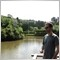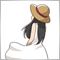# DRAW_SECTION and DRAW_LINE in the same indicator729

Hi for all!

I want to create a custom zigzag with a line (like MA). I tought to use a DRAW_SECTION (for zigzag) and a DRAW_LINE (for my line).

All looks fine, but when I try to run, there is no horizontal line and appear several vertical lines. I already developed other indicators using DRAW_SECTION and using DRAW_LINE. But it's my first time using both in the same indicator. What I'm missing?

Result:

Code: It is the mql5 zigzag example code with the line indicator structure

```#property copyright "mql5"
#property version   "1.00"
#property indicator_chart_window
#property indicator_buffers 4
#property indicator_plots   2
//--- plot ZigZag
#property indicator_label1  "ZigZag"
#property indicator_type1   DRAW_SECTION
#property indicator_color1  clrRed
#property indicator_style1  STYLE_SOLID
#property indicator_width1  1
//--- plot Label2
#property indicator_label2  "Line"
#property indicator_type2   DRAW_LINE
#property indicator_color2  clrRed
#property indicator_style2  STYLE_DOT
#property indicator_width2  1

//--- input parameters
input int InpDepth    =12;  // Depth
input int InpDeviation=5;   // Deviation
input int InpBackstep =3;   // Back Step
//--- indicator buffers
double         ZigZagBuffer[];      // main buffer
double         HighMapBuffer[];     // ZigZag high extremes (peaks)
double         LowMapBuffer[];      // ZigZag low extremes (bottoms)
double         LineBuffer[];

int       ExtRecalc=3;         // number of last extremes for recalculation

enum EnSearchMode {
Extremum=0, // searching for the first extremum
Peak=1,     // searching for the next ZigZag peak
Bottom=-1   // searching for the next ZigZag bottom
};
//+------------------------------------------------------------------+
//| Custom indicator initialization function                         |
//+------------------------------------------------------------------+
int OnInit(){
//--- indicator buffers mapping
SetIndexBuffer(0,ZigZagBuffer,INDICATOR_DATA);
SetIndexBuffer(1,HighMapBuffer,INDICATOR_CALCULATIONS);
SetIndexBuffer(2,LowMapBuffer,INDICATOR_CALCULATIONS);
SetIndexBuffer(3,LineBuffer,INDICATOR_DATA);
//--- set short name and digits
string short_name=StringFormat("ZigZag(%d,%d,%d)",InpDepth,InpDeviation,InpBackstep);
IndicatorSetString(INDICATOR_SHORTNAME,short_name);
PlotIndexSetString(0,PLOT_LABEL,short_name);
IndicatorSetInteger(INDICATOR_DIGITS,_Digits);
//--- set an empty value
PlotIndexSetDouble(0,PLOT_EMPTY_VALUE,0.0);
PlotIndexSetDouble(3,PLOT_EMPTY_VALUE,0.0);
return(INIT_SUCCEEDED);
}

//+------------------------------------------------------------------+
//| ZigZag calculation                                               |
//+------------------------------------------------------------------+
int OnCalculate(const int rates_total,
const int prev_calculated,
const datetime &time[],
const double &open[],
const double &high[],
const double &low[],
const double &close[],
const long &tick_volume[],
const long &volume[],

if(rates_total<100) return(0);

int    i=0;
int    start=0,extreme_counter=0,extreme_search=Extremum;
int    shift=0,back=0,last_high_pos=0,last_low_pos=0;
double val=0,res=0;
double curlow=0,curhigh=0,last_high=0,last_low=0;

if(prev_calculated==0){
ArrayInitialize(ZigZagBuffer,0.0);
ArrayInitialize(HighMapBuffer,0.0);
ArrayInitialize(LowMapBuffer,0.0);
ArrayInitialize(LineBuffer,0.0);
start=InpDepth;
}

if(prev_calculated>0){
i=rates_total-1;
//--- searching for the third extremum from the last uncompleted bar
while(extreme_counter<ExtRecalc && i>rates_total-100){
res=ZigZagBuffer[i];
if(res!=0.0)
extreme_counter++;
i--;
}
i++;
start=i;

//--- what type of exremum we search for
if(LowMapBuffer[i]!=0.0){
curlow=LowMapBuffer[i];
extreme_search=Peak;
} else {
curhigh=HighMapBuffer[i];
extreme_search=Bottom;
}
//--- clear indicator values
for(i=start+1; i<rates_total && !IsStopped(); i++){
ZigZagBuffer[i] =0.0;
LowMapBuffer[i] =0.0;
HighMapBuffer[i]=0.0;
LineBuffer[i]=0.0;
}
}

//--- searching for high and low extremes
for(shift=start; shift<rates_total && !IsStopped(); shift++){
//--- low
val=low[Lowest(low,InpDepth,shift)];
if(val==last_low)
val=0.0;
else {
last_low=val;
if((low[shift]-val)>InpDeviation*_Point)
val=0.0;
else {
for(back=1; back<=InpBackstep; back++){
res=LowMapBuffer[shift-back];
if((res!=0) && (res>val))
LowMapBuffer[shift-back]=0.0;
}
}
}
if(low[shift]==val)
LowMapBuffer[shift]=val;
else
LowMapBuffer[shift]=0.0;
//--- high
val=high[Highest(high,InpDepth,shift)];
if(val==last_high)
val=0.0;
else {
last_high=val;
if((val-high[shift])>InpDeviation*_Point)
val=0.0;
else{
for(back=1; back<=InpBackstep; back++){
res=HighMapBuffer[shift-back];
if((res!=0) && (res<val))
HighMapBuffer[shift-back]=0.0;
}
}
}
if(high[shift]==val)
HighMapBuffer[shift]=val;
else
HighMapBuffer[shift]=0.0;
}

//--- set last values
if(extreme_search==0){
last_low=0.0;
last_high=0.0;
} else {
last_low=curlow;
last_high=curhigh;
}

//--- final selection of extreme points for ZigZag
for(shift=start; shift<rates_total && !IsStopped(); shift++){
res=0.0;
LineBuffer[shift]=open[shift];  //<--- always pass by here
switch(extreme_search){
case Extremum:
if(last_low==0.0 && last_high==0.0){
if(HighMapBuffer[shift]!=0){
last_high=high[shift];
last_high_pos=shift;
extreme_search=Bottom;
ZigZagBuffer[shift]=last_high;
res=1;
}
if(LowMapBuffer[shift]!=0.0){
last_low=low[shift];
last_low_pos=shift;
extreme_search=Peak;
ZigZagBuffer[shift]=last_low;
res=1;
}
}
break;
case Peak:
if(LowMapBuffer[shift]!=0.0 && LowMapBuffer[shift]<last_low && HighMapBuffer[shift]==0.0){
ZigZagBuffer[last_low_pos]=0.0;
last_low_pos=shift;
last_low=LowMapBuffer[shift];
ZigZagBuffer[shift]=last_low;     // \$\$\$\$\$\$\$\$\$\$\$\$\$\$\$\$\$\$\$\$\$\$\$\$\$\$\$\$\$\$\$\$\$\$\$\$\$\$\$\$\$\$\$\$\$\$\$\$\$\$\$\$\$\$\$\$\$\$\$\$\$\$\$\$\$\$\$\$\$\$\$
res=1;
}
if(HighMapBuffer[shift]!=0.0 && LowMapBuffer[shift]==0.0){
last_high=HighMapBuffer[shift];
last_high_pos=shift;
ZigZagBuffer[shift]=last_high;     // \$\$\$\$\$\$\$\$\$\$\$\$\$\$\$\$\$\$\$\$\$\$\$\$\$\$\$\$\$\$\$\$\$\$\$\$\$\$\$\$\$\$\$\$\$\$\$\$\$\$\$\$\$\$\$\$\$\$\$\$\$\$\$\$\$\$\$\$\$\$\$
extreme_search=Bottom;
res=1;
}
break;
case Bottom:
if(HighMapBuffer[shift]!=0.0 && HighMapBuffer[shift]>last_high && LowMapBuffer[shift]==0.0){
ZigZagBuffer[last_high_pos]=0.0;
last_high_pos=shift;
last_high=HighMapBuffer[shift];
ZigZagBuffer[shift]=last_high;     // \$\$\$\$\$\$\$\$\$\$\$\$\$\$\$\$\$\$\$\$\$\$\$\$\$\$\$\$\$\$\$\$\$\$\$\$\$\$\$\$\$\$\$\$\$\$\$\$\$\$\$\$\$\$\$\$\$\$\$\$\$\$\$\$\$\$\$\$\$\$\$
}
if(LowMapBuffer[shift]!=0.0 && HighMapBuffer[shift]==0.0){
last_low=LowMapBuffer[shift];
last_low_pos=shift;
ZigZagBuffer[shift]=last_low;     // \$\$\$\$\$\$\$\$\$\$\$\$\$\$\$\$\$\$\$\$\$\$\$\$\$\$\$\$\$\$\$\$\$\$\$\$\$\$\$\$\$\$\$\$\$\$\$\$\$\$\$\$\$\$\$\$\$\$\$\$\$\$\$\$\$\$\$\$\$\$\$
extreme_search=Peak;
}
break;
default:
return(rates_total);
}
}

return(rates_total);
}
//+------------------------------------------------------------------+
//|  Search for the index of the highest bar                         |
//+------------------------------------------------------------------+
int Highest(const double &array[],const int depth,const int start){
if(start<0) return(0);

double max=array[start];
int    index=start;

for(int i=start-1; i>start-depth && i>=0; i--){
if(array[i]>max){
index=i;
max=array[i];
}
}
return(index);
}
//+------------------------------------------------------------------+
//|  Search for the index of the lowest bar                          |
//+------------------------------------------------------------------+
int Lowest(const double &array[],const int depth,const int start){
if(start<0) return(0);

double min=array[start];
int    index=start;

for(int i=start-1; i>start-depth && i>=0; i--){
if(array[i]<min){
index=i;
min=array[i];
}
}
return(index);
}
//+------------------------------------------------------------------+```841

You need to map buffer 0 to property 1 and buffer 1 to property 2.

```SetIndexBuffer(0,ZigZagBuffer,INDICATOR_DATA);
SetIndexBuffer(1 ,LineBuffer,INDICATOR_DATA);```729